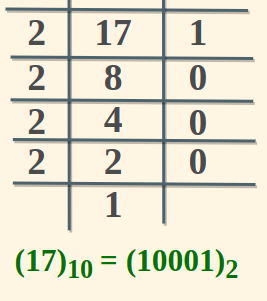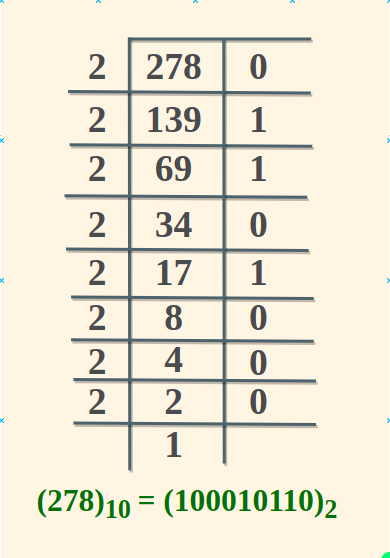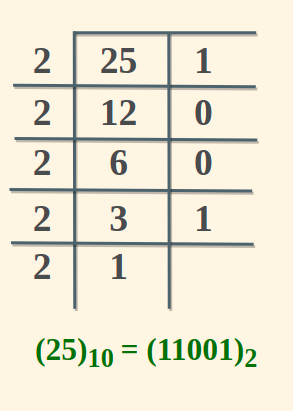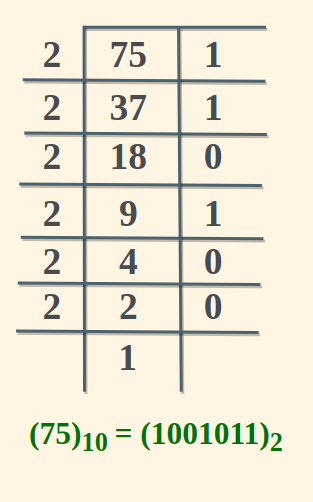Open In App

# Decimal to Binary Converter

Decimal to Binary Converter is a free online tool to convert Decimal to Binary. Converting between Decimal to Binary is a common task in the everyday life of cs students or programmers. So, GeeksforGeeks provides a free user-friendly and efficient online decimal to binary Conversion tool to simplify this process and ensure accuracy. It is a fast, easy-to-use general purpose calculator that can be used in any field such as Academics etc. Furthermore, it also helps students and working professionals to solve a wide range of day-to-day problems.

## How to use Decimal to Binary Calculator?

We can easily use the decimal-to-binary calculator by following the steps discussed below,

Step 1: Enter the given value in the decimal input field.

Step 2: Click on the convert button to convert the decimal value into the binary value.

Step 3: The value shown as the result is the required value in the binary form.

## Decimal to Binary Conversion

Before learning how to convert decimal to binary in a number system, let’s first understand what a decimal number system is and what is a binary number system.

### Decimal Number System

The number system that has a base value of 10 is called Decimal Number System. Decimal Numbers are consist of the following digits: 0, 1, 2, 3, 4, 5, 6, 7, 8, 9.

### Binary Number System

A binary Number System is a base-2 number system that uses two states 0 and 1 to represent a number. For example: 01, 111, etc.

All the decimal numbers have their corresponding binary numbers. These binary numbers are used in computer applications and used for programming or coding purposes. This is because binary digits, 0 and 1 are only understood by computers.

### Steps for Decimal to Binary Conversion

For converting Decimal numbers into binary numbers, use different methods such as formula, division methods, and so on. Here, use the remainder formula. Steps to convert decimal number to binary number using decimal to the binary formula are as follow,

Step 1: Divide the given decimal number by 2, and find the remainder (Ri).

Step 2: Now divide the quotient (Qi) that is obtained in the above step by 2, and find the remainder.

Step 3: Repeat the above steps 1 and 2, until 0 is obtained as a quotient.

Step 4: Write down the remainder in the following manner: the last remainder is written first, followed by the rest in the reverse order (Rn, R(n – 1) …. R1). thus binary conversion of the given decimal number will be obtained.

Let’s understand the above steps with the help of an example

Example: Convert 17 to Binary Form

Solution:

Following the above steps we will divide 17 by 2 successively. The division process is shown the image added below:Hence the Binary Equivalent of 17 is 10001.

Also Check: Binary to Decimal Converter

## Decimal to Binary Conversion Table

The common numbers in Decimal system and their corresponding binary form is tabulated below:

Decimal Number

Binary Number

00
11
210
311
4100
5101
6110
7111
81000
91001
101010
111011
121100
131101
141110
151111
1610000
1710001
1810010
1910011
2010100
2110101
2210110
2310111
2411000
2511001
2711011
2811100
2911101
3011110
3111111
32100000
641000000
12810000000
256100000000

## Conclusion

In Conclusion, Decimal to Binary Calculator is a free online tool prepared by GeekforGeeks that converts the given value of the decimal number into the value of the binary number (0,1). It is a fast and easy-to-use tool that helps students solve various problems.

Also Check

## Decimal to Binary Converter Examples

Example 1: What is equivalent value of (278)10 in Binary?

Solution:

We have 278 in Decimal. To Convert in Binary we will divide 278 successively by 2.Hence, (278) in decimal is equivalent to (100010110) in binary.

Example 2: Convert (25)10 in Binary

Solution:

We have 25 in decimal. To convert 25 in binary we will divide 25 by 2 successivelyHence, the Binary Equivalent of 25 is 11001

Example 3: What is the value of 75 in Binary?

Solution:

We have 75 in decimal. To convert 75 in binary we will divide 25 by 2 successivelyHence, the Binary Equivalent of 75 is 1001011

## Convert Decimal to Binary

Q1: Convert 248 in Binary

Q2: Convert 575 in Binary

Q3: What is Binary equivalent of 49

Q4: Convert (56)10 to (….)2

Q5: What is the Binary Form of 95

## FAQs on Decimal to Binary Conversion

### 1. Using Decimal to Binary Formula, convert 3 decimal into a binary number.

Using decimal to binary formula,

Step 1: Divide the number by 3, find the remainder.

3 ÷ 2 gives Q1 = 1, R1 = 1

Step 2: Divide Q1 by 2, find the remainder.

1 ÷ 2 gives Q2 = 0, R2 = 1

Step 3: Write down the remainder in the following manner: the last remainder is written first, followed by the rest in the reverse order (Rn, R(n – 1) …. R1), this is the binary conversion of the given decimal number: 11

Answer: Hence, 3 as binary is (11)2

### 2. Using Decimal to Binary Formula, convert 5 Decimal into a Binary number.

Using decimal to binary formula,

Step 1: Divide the number by 5, find the remainder.

5 ÷ 2 gives Q1 = 1, R1 = 1

Step 2: Divide Q1 by 2, find the remainder.

2 ÷ 2 gives Q2 = 1, R2 = 0

Step 3: Divide Q2 by 2, find the remainder.

1 ÷ 2 gives  Q3 = 0, R3 = 1

Step 4: Write down the remainder in the following manner: the last remainder is written first, followed by the rest in the reverse order (Rn, R(n – 1) …. R1), this is the binary conversion of the given decimal number: 101

Answer: Hence, 5 as binary is (101)2

### 3. Using Decimal to Binary formula, convert 8 Decimal into a Binary number.

Using decimal to binary formula,

Step 1: Divide the number by 8, find the remainder.

8 ÷ 2 gives Q1 = 2, R1 = 0

Step 2: Divide  Q1 by 2, find the remainder.

4 ÷ 2 gives Q2 = 1, R2 = 0

Step 3: Divide  Q2 by 2, find the remainder.

2 ÷ 2 gives Q3 = 0, R3 = 0

Step 4: Divide  Q3 by 2, find the remainder.

1 ÷ 2 gives Q4 = 0, R4 = 1

Step 5: Write down the remainder in the following manner: the last remainder is written first, followed by the rest in the reverse order (Rn, R(n – 1) …. R1), this is the binary conversion of the given decimal number: 1000

Answer: Hence, 8 as binary is (1000)2

### 4. How to Convert Decimal Numbers to Binary Numbers?

To convert decimal numbers to binary, repeatedly divide the decimal number by 2, noting remainders. Write down the remainders in reverse order to get the binary representation. For example, 25 in binary is 11001.